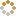﻿ N-B-U-T Online Judge ::  Execute HVM
• ###  Execute HVM

• 时间限制: 1000 ms　内存限制: 65535 K
• 问题描述
• HVM is short for Hack Virtual Machine. It's a tiny script.

Maybe most of you know it. If you don't know, you can go http://www.hacker.org/hvm for more information.

This problem sounds very simple, just to write a simple HVM executor.
The original HVM is not written by C or C++, so it's a little different. If abs (a) > abs (b) (a, b != 0) and a * b < 0, then b / a will be -1. But in C/C++, it will be 0. So you have to do something to adapt it.

• 输入
• This problem has several cases, input until EOF.
The first line of each case has one integer N (0 <= N <= 16384), indicates the number of initialized memory buffer.
If N is not 0, it follows a line has N integer(s). The ith number indicates MemoryBuffer[i].
Next line contains a string (no longer then 2048), indicates the HVM Command. You can consider that all of the data is valid.
• 输出
• For each case, you should output the trace box and the result that the command will print.
Each step of the command except empty command (' ') would have a trace. The format is:
Command [Stack element y, Stack element y – 1, ... Stack top]
Notice that there's a blank space after ','.
• 样例输入
• ```0
123ppp
```
• 样例输出
• ```1 
2 [1, 2]
3 [1, 2, 3]
p [1, 2]
p 
p []
321
```
• 提示
• `无`
• 来源
• `XadillaX@NBUT`
• 操作
﻿